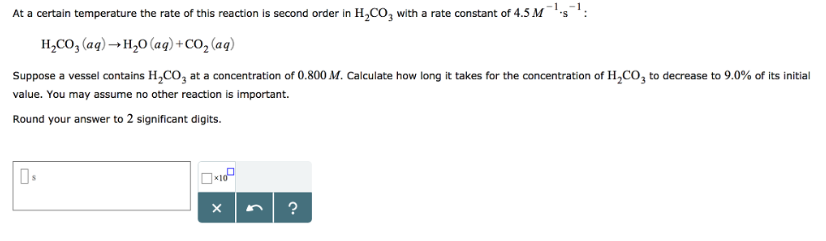# Problem: At a certain temperature the rate of this reaction is second order in H2CO3 with a rate constant of 4.5M-1s-1. H2CO3 (aq) → H2O (aq) + CO2 (aq)Suppose a vessel contains H2CO3 at a concentration of 0.800 M. Calculate how long it takes for the concentration of H2CO3 to decrease to 9.0% of its initial value. You may assume no other reaction is important. Round your answer to 2 significant digits.

###### FREE Expert Solution
86% (473 ratings)###### Problem Details

At a certain temperature the rate of this reaction is second order in H2CO3 with a rate constant of 4.5M-1s-1

H2CO3 (aq) → H2O (aq) + CO2 (aq)

Suppose a vessel contains H2CO3 at a concentration of 0.800 M. Calculate how long it takes for the concentration of H2CO3 to decrease to 9.0% of its initial value. You may assume no other reaction is important.

Round your answer to 2 significant digits.Frequently Asked Questions

What scientific concept do you need to know in order to solve this problem?

Our tutors have indicated that to solve this problem you will need to apply the Integrated Rate Law concept. You can view video lessons to learn Integrated Rate Law. Or if you need more Integrated Rate Law practice, you can also practice Integrated Rate Law practice problems.

What professor is this problem relevant for?

Based on our data, we think this problem is relevant for Professor McCartney's class at TAMU.